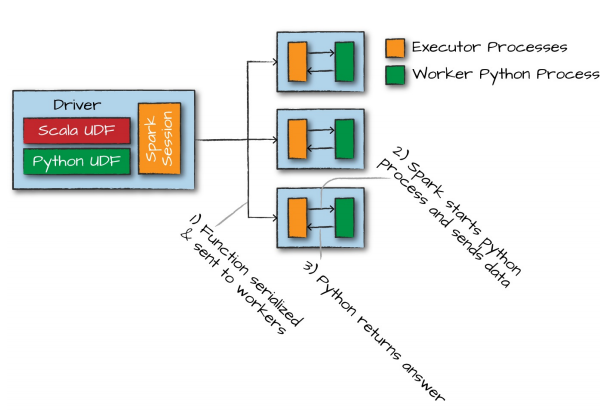# 自定义函数,Spark,用户,UDF

### UDF介绍

spark的强大功能之一就是可以让用户自定义函数。 用户自定义函数（UDF）让用户可以使用Scala或Python编写自己的自定义 转换（Transformation)操作 ，甚至可以使用外部库。
UDF可以将一个或多个列作为输入，同时也可以返回一个或多个列。Spark的UDF允许使用多种不同的编程语言编写。 这些函数只是描述了（一个接一个地）处理数据记录的方法 。 默认情况下，这些函数被注册为SparkSession或者Context的临时函数。

### 编写UDF函数的步骤

#### 第一步：设计一个实际的函数

``````//  先创建一个DF
val udfExampleDF = spark.range(5).toDF("num")
//  第一步：设计一个实际的函数
//  注意：这个自定义函数有指定接受参数类型，和返回类型
def power3(number:Double): Double = number * number * number
``````

#### 第二步：在Spark上注册UDF

Spark将在驱动进程上序列化该函数，并将它通过网络传递到所有执行进程，无论使用何种语言都是这个过程。

``````//    第二步，在spark上注册该UDF
val power3UDF = udf(power3(_: Double): Double)
//    val power3UDF = udf(power3 _)    //简写形式
``````#### 第三步 像使用其他DataFrame函数一样使用UDF

``````    udfExampleDF.select(power3UDF(col("num"))).show()
``````

#### 完整代码

``````import org.apache.spark.sql.{DataFrame, SparkSession}
import org.apache.spark.sql.functions.{col, udf}

object Test {

def main(args: Array[String]): Unit = {

val spark = new SparkSession.Builder()
.config("spark.testing.memory", "471859200")
.master("local[" +
"4]")
.getOrCreate()

val udfExampleDF: DataFrame = spark.range(5).toDF("num")

println(udfExampleDF.schema)

val power3UDF = udf(power3 _)

udfExampleDF.select(power3UDF(col("num"))).show()
}

def power3(number:Long): Long = number * number * number
}
``````Python Free

QQ：417803890